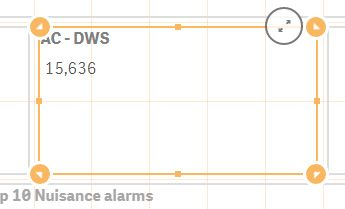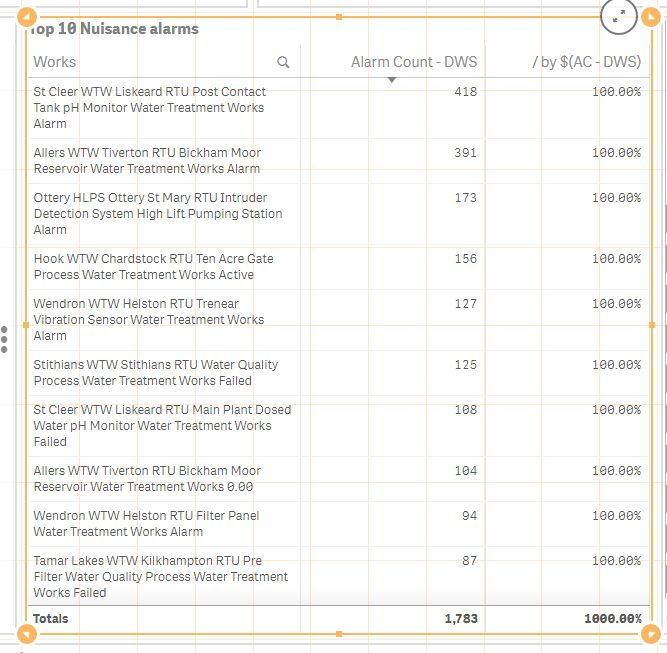# New to Qlik Sense

Discussion board where members can get started with Qlik Sense.

Contributor

## Measure not dividing by variable amount

Hi there,

I'm trying to calculate percentage values in a table.

I've created a variable called (AC - DWS) which calculates the total number of records for a measure. This calculates and I can output the results to a text box:I'm using this variable to divide by the total records for a particular dimension., If I manually add the result as a number, the division works:If I use the result of the variable, it doesn't:Any idea why? It also doesn't work if I add the calculation used to get the variable value:

Count({1<[Ops Function]={'Drinking Water'}, DataSource={'Alarms'}, [Alarm Status]={3}, Date={'>=\$(=Date(\$(vTodaysDate)))'}>} AlarmCount)

Tags (2)
1 Solution

Accepted Solutions
HighlightedMVP

## Re: Measure not dividing by variable amount

Did you try adding a '=' before your Count function in the variable?

=Count({1<[Ops Function]={'Drinking Water'}, DataSource={'Alarms'}, [Alarm Status]={3}, Date={'>=\$(=Date(\$(vTodaysDate)))'}>} AlarmCount)

7 Replies
Valued Contributor II

## Re: Measure not dividing by variable amount

Hi Neil,

Can you please tell me or show me the expression how you are creating the variable ?

HighlightedMVP

## Re: Measure not dividing by variable amount

Did you try adding a '=' before your Count function in the variable?

=Count({1<[Ops Function]={'Drinking Water'}, DataSource={'Alarms'}, [Alarm Status]={3}, Date={'>=\$(=Date(\$(vTodaysDate)))'}>} AlarmCount)

Contributor

## Re: Measure not dividing by variable amount

Hi Sunny,

Thank you - that worked. The odd thing is that it had already been working up till we had 3.0 installed, so perhaps the syntax has been tightened on variable creation.MVP

## Re: Measure not dividing by variable amount

I think this blogs talk about what we just saw

The Magic of Variables

Contributor

## Re: Measure not dividing by variable amount

I'll read that one thoroughly! Thank you for all your help (again!)MVP

## Re: Measure not dividing by variable amount

No problem at allContributor

## Re: Measure not dividing by variable amount

Hi Rohit,

All solved thank you - Sunny (posts below) showed me the way....

Thank you for posting tho!

Neil